Courses

# Test: Design For Static And Dynamic Load (Level - 3)

## 20 Questions MCQ Test Machine Design | Test: Design For Static And Dynamic Load (Level - 3)

Description
This mock test of Test: Design For Static And Dynamic Load (Level - 3) for Mechanical Engineering helps you for every Mechanical Engineering entrance exam. This contains 20 Multiple Choice Questions for Mechanical Engineering Test: Design For Static And Dynamic Load (Level - 3) (mcq) to study with solutions a complete question bank. The solved questions answers in this Test: Design For Static And Dynamic Load (Level - 3) quiz give you a good mix of easy questions and tough questions. Mechanical Engineering students definitely take this Test: Design For Static And Dynamic Load (Level - 3) exercise for a better result in the exam. You can find other Test: Design For Static And Dynamic Load (Level - 3) extra questions, long questions & short questions for Mechanical Engineering on EduRev as well by searching above.
QUESTION: 1

Solution:
QUESTION: 2

### A S-N curve for steel is shown in figure. The corrected endurance limit is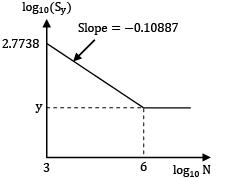Sy: Yield strength in MPa.

Solution:

Equation of line,

(y - 2.7738) = -0.10887(x - 3)

at x = 6,y = 2.44719

Sf = (10)y = 280.0206 MPa

*Answer can only contain numeric values
QUESTION: 3

### A forged steel bar of 50 mm diameter, is subjected to a reversed bending stress of 250N/mm2 . The bar is made of steel 40 C8 mm (Sut = 600 N/mm2)and the corrected endurance limit of the bar is 101N/mm2 , then life of the steel bar will be __________ cycles.

Solution:

Sf = 250 N/mm2

Sut = 600 N/mm2

Se = 101N/mm2

The S-N curve consists of a straight line (AB) drawn from 0.9 Sut at 103 cycles to at cycles at Se = 106 on log graph

0.9 sut = 0.9 x 600 = 540 N/mm2

log10 (0.9 sut) = log10(540) = 2.7323

log10(Se) = log10(101) = 20043

log10(sf) = log10(250) = 2.3979

log10(103) = 3

log10 (106) = 6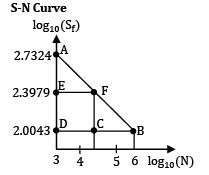Fatigue life of bar, from figure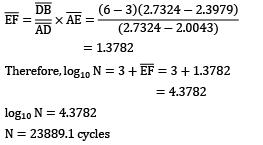= 4.3782

log10 N = 4.3782

N = 23889.1 cycle

Therefore,log10 N = 3 + EF = 3 + 1.3782

*Answer can only contain numeric values
QUESTION: 4

A rotating bar of steel (Sut = 600N/mm2) is subjected to a completely reversed bending stress. The corrected endurance limit of the bar is 315N/mm2 . The fatigue strength (in N/mm2) of the bar for a life of 70000 cycles is __________

Solution:

Given: sut = 630 N/mm2; se = 315 N/mm2

N = 70000 cycles

Now,log10(0.9 Sut) = log10(567) = 2.7536

Log10 (se) = log10(315) = 2.4983

log10(N) = log10(70000) = 4.8451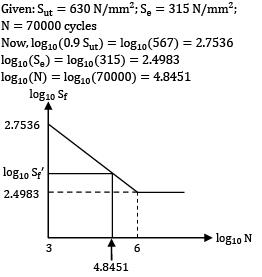So, from S-N diagram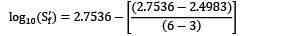x (4.8451 - 3)

log10 (s’f) = 2.5966

S’f = (10)2.5966 = 395 N/mm2

QUESTION: 5

When st!ess and Young’s Modulus of Elasticity E remain constant, the energyabsorbing capacity of part subjected to dynamic axial forces, is a function of its

Solution:

Strain energy is given by,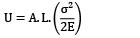Where,

A = Area of cross-section

L = Length of the component

Where and E remaining constant,

∴U is proportional to (A.L.) which is volume.

QUESTION: 6

Fatigue strength of a rod subjected to cyclic axial force is less than that of a rotating beam of the same dimensions subjected to steady lateral force. What is the reason?

Solution:

In case of axial load, the stress distribution will be uniform and every point in the rod will be subjected to same stress. But in case of bending stress (due to lateral force) every point is not subjected to same stress, as the bending stress distribution is linear.

*Answer can only contain numeric values
QUESTION: 7

A flat plate is subjected to a tensile force of 10kN. The permissible stress in the plate is 100N/mm2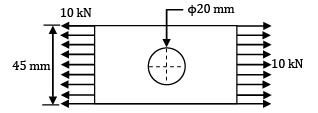The stress concentration factor of hole section is 2.1. The thickness of plate is __________ mm.

Solution:

Tensile stress in hole section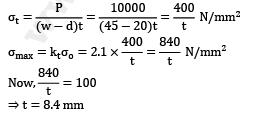⇒ t = 8.4 mm

QUESTION: 8

A component is subjected to a dynamic stress given by 200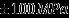a. The material is ductile and has the following properties

Sut = 600MPa, Syt = 400MPa, Se = 300MPa

The factor of safety based on Goodman’s and Soderberg’s criteria are respectively

Solution:

σmax = 300 MPa,σmin= 100 MPa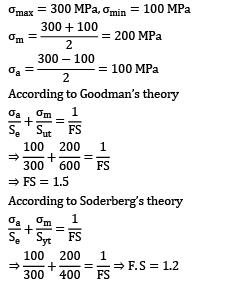According to Goodman’s theory⇒ FS = 1.5

According to Soderberg’s theory*Answer can only contain numeric values
QUESTION: 9

A ductile material having an endurance limit of 200N/mm2 and the yield limit is 300N/mm2 , is stressed under variable load. The maximum and minimum stressed are 150N/mm2 and 50N/mm2 . The fatigue stress concentration factor is 1.3. The factor of safety for this loading is __________.

Solution:

Given

σmax = 150 MPa

σmin = 50 MPa

Since, the material is ductile

Apply Soderberg Equation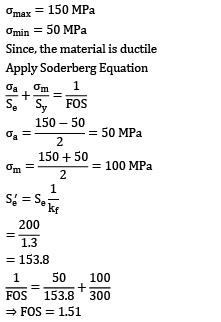= 153.8⇒ FOS = 1.51

*Answer can only contain numeric values
QUESTION: 10

A plate made of grey cast iron in hot-rolled and normalized condition. It is subjected to a axial load of (1+sin2x) . The amplitude ratio will be __________ (upto two decimal places).

Solution:

The graph of (1 = sin2x)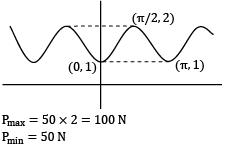Pmax = 50 x 2 = 100 N

Pmin = 50 N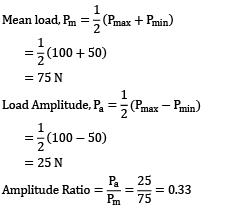= 75 N= 25 NQUESTION: 11

A component is subjected to a flexural stress which fluctuates between 310N/mm2 and -160N/mm2 . If endurance strength is half of the ultimate strength, the minimum ultimate strength according to Gerber relation is (Factor of safety = 2)

Solution:

Given,

σmax = 310 MPa

σmin = -160 MPa

Se = 0.5 sut

N = 2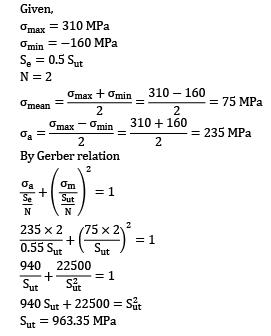By Gerber relationSut = 963.35 MPa

QUESTION: 12

A cantilever beam is subjected to completely reversed bending moment of 200 Nm. The log S log N diagram for the beam is shown in the figure. The diameter d of the beam for a life of 105 cycles is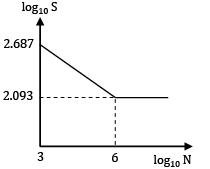Solution: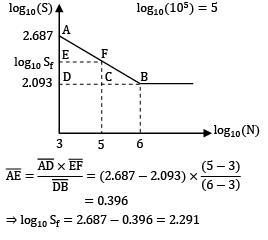= 0.396

⇒log10 sf = 2.687 - 0.396 = 2.291

Sf = 195.43 N/mm2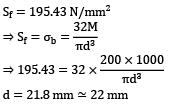d = 21.8 mm ≃ 22 mm

QUESTION: 13

A steel connecting rod is subjected to a completely reverse axial load of 100 kN. For the steel material Su = 1100MP a, Sy = 930MPa,Se = 0.5, Su.load factor 0.85, Size factor 0.85 and su!face finish factor = 0.76. What is the suitable size of the rod using a factor of safety 2?

(A) 30 mm

(B) 45 mm

(C) 60 mm

(D) 75 mm

Solution:

Given,

Completely reversed axial load(P) = 100KN

Ultimate strength sCH= 1100 MPa

Yield strength Syt = 930 MPa

Endurance strength se = 0.5 sut

Load factor (ka) = 0.85

Size factor (kb) = 0.85

Surface finish factor (kc) 0.76

Factor safety (N) = 2

Corrected endurance limit S’e = Sekakbkc

S’e = 0.5 x 1100 x 0.85 x 0.85 x 0.76

S’e = 302.005 MPa

For completely reversed loading,

Mean stress σm = 0

Stress Amplitude σa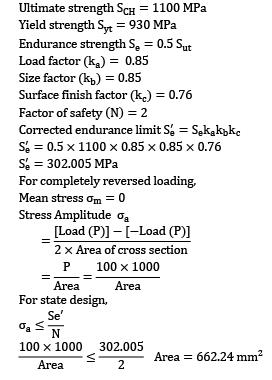For state design*Answer can only contain numeric values
QUESTION: 14

A shaft of diameter d is subjected to a torque varying between 100 N.m to 500 N.m. Stress concentration factor due to keyway is 1.5.

Taking factor of safety 2 yield strength 300 MPa and endurance limit of 200 MPa. Correction factor for torsion is . , Surface finish factor for torsion is 0.6 and Surface finish factor is 0.85, and size factor is 0.82 The diameter of the shaft (mm) will be __________

Solution: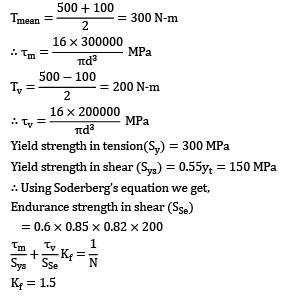Yield strength in tension(Sy) = 300 MPa

Yield strength in shear (Sys) = 0.55yt = 150 MPa

∴Using Soderberg’s equation we get,

Endurance strength in shear (SSe)

= 0.6 x 0.85 x 0.82 x 200Kf = 1.5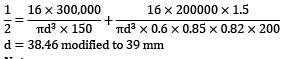d = 38.46 modified to 39 mm

Note:

As per the recent observation a component subjected to a varying torque has negligible effect due to mean shear stress. Hence, the design may be based only on the variable shear stress.

QUESTION: 15

A uniform bar having a machined surface is subjected to an axial load varying from 400 kN to 150 kN. The material of the bar has Su= 630 MPa,kc = 0.7 and kf= 1.42 Using Goodman’s Criteria and factor of safety 1.5, The diameter of the rod is __________ mm, Where, Su = ultimate tensile strength,kc = surface finish factor, kf = fatigue st!ess concentration factor

Solution: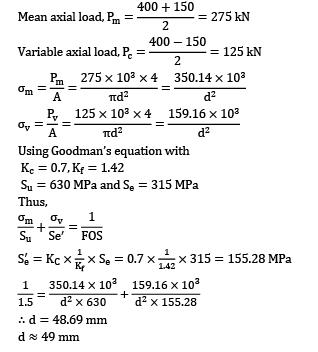Using Goodman’s equation with

Kc = 0.7, Kf = 1.42

Su = 630 MPa and Se = 315 MPa

Thus,∴d = 48.69 mm

d ≈ 49 mm

Question_type5

QUESTION: 16

A bar of circular cross-section is subjected to alternating tensile forces varying from a minimum of 200 kN to a maximum of 500 kN. It is to be manufactured of a material with an ultimate tensile strength of 900 MPa and an endurance limit of 700 MPa. Using safety factors of 3.5 related to ultimate tensile strength and 4 related to endurance limit and a stress concentration factor of 1.65 for fatigue load. Using Goodman straight line as basis for design, the diameter of circular bar is

Solution: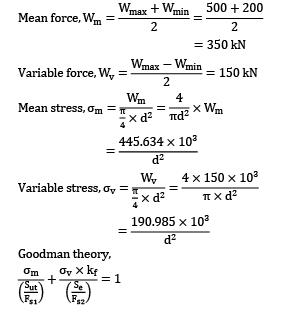= 350 kNGoodman theory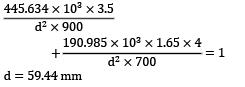d = 59.44 mm

QUESTION: 17

A pulley is supported midway between the two bearings spaced 400 mm apart on a shaft rotating at 1400 rpm. The tensions acting vertically downwards on the tight and slack side of the belt are 1000 N and 500 N respectively. The material of the shaft is 30 C8 steel with Se= 280MPa,Su = 600MPa,

kf = 1.85 FOS = 3, the diameter of the shaft

Solution:

Given

L = 400 mm,

N = 1400 rpm,

T1 = 1000 N,

T2 = 500 N,

S’e 280 MPa, Sut = 600 MPa

Kt = 1.85, F. S = 3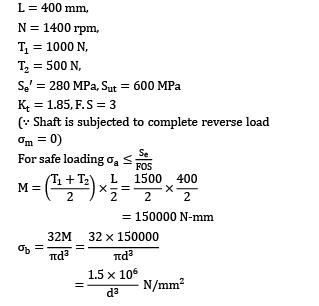= 150000 N-m(∵Shaft is subjected to complete reverse load σm = 0)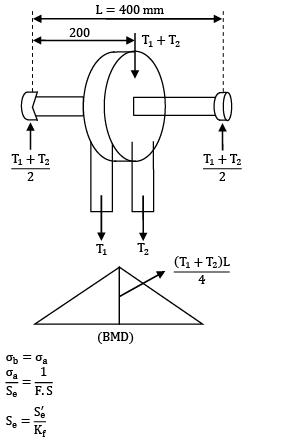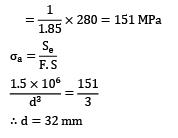∴ d = 32 mm

QUESTION: 18

Bending stress in a machine component fluctuates between a tensile stress of 280 MPa and compressive stress for 140 MPa. The factor of safety is 1.75. The yield strength is equal to 55% of ultimate tensile strength and endurance strength is 50% of ultimate tensile strength. What should be the minimum ultimate tensile strength to carry this fluctuation indefinitely according to Soderberg’s criterion?

(A) 1155 MPa

(B) 955 MPa

(C) 725 MPa

(D) 575 MPa

Solution:

Given, Fs = 1.75

σmax = 280 MPa, σmin = -140 MPa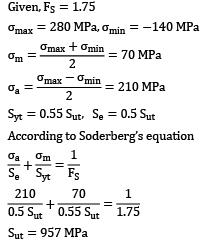Syt = 0.55 Sut,, Se = 0.5 Sut

According to Soderberg’s equationSut = 957 MPa

QUESTION: 19

A steel plate is subjected to a completely reversed axial load of 40 kN and it has 10 mm diameter hole. Width of the plate is 60 mm. If surface finish factor (ka) = 0.65 size factor (kb) = 0.75 Reliability factor (kc) = 0.789, Notch sensitivity (q) =.0.82 and theoretical stress concentration factor (kt) = 2.5. The ultimate tensile strength of the material (Sut) is 400 MPa. If the factor of safety is 2. What will be the thickness of the plate for infinite life (Take endurance limit as 50% of ultimate tensile strength and correction factor for axial load is 0.82)

Solution: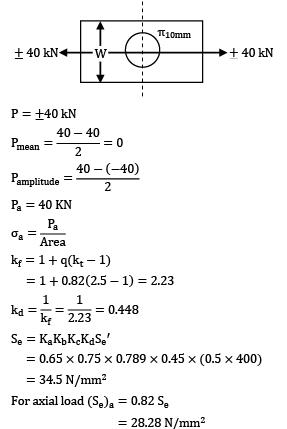P = 土40 kNPa = 40 kNkf = 1 + q(kt - 1)

= 1 + 0.82(2.5 - 1)se= KaKbKcKdSe

= 0.65 x 0.75 x 0.789 x 0.45 x (0.5 x 400)

= 34.5 N/mm2

For axial load (Se)a = 0.82 Se

= 28.28 N/mm2

kf = 1 + q(kt - 1)

= 1 + 0.82(2.5 - 1)

For safe loading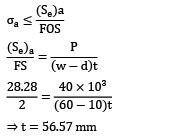⇒ t = 56.57 mm

*Answer can only contain numeric values
QUESTION: 20

A circular bar of 500 mm length is supported by a hinge at its two ends. It is acted upon by a central cyclic load having a minimum value of 20 kN and a maximum value of 50 kN. The material properties of bar are given by: Ultimate strength of 650 MPa, yield strength of 500 MPa and endurance strength of 350 MPa. Taking a factor of safety of 1.5, size effect of 0.85, surface finish factor of 0.9, the diameter of the bar as per Goodman’s criteria is __________ mm

(A) 59

(B) 60.50

Solution:

Given

l = 500 mm

Wmin =20 KN, Wmax =50 KN, F.S. = 1.5

Ka = 0.9, Kb = 0.85, Sut = 650 MPa,

Syt = 500 MPa, Se = 350 MPa

Let d = diameter of the bar in mm

Maximum bending moment is given by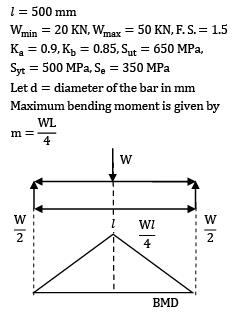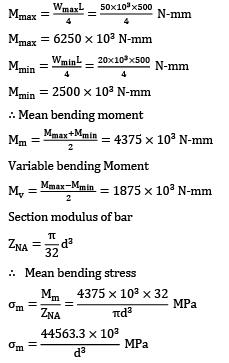Mmax = 6250 x 103 N-mMmin = 2500 x 103 N-mm

∴Mean bending momentVariable bending momentSection modulus of bar∴ Mean bending stressVariable bending stress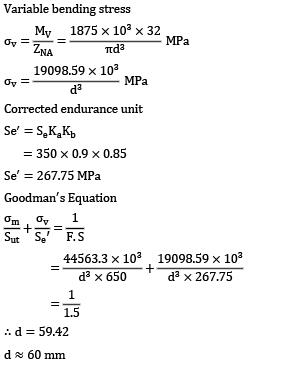Corrected endurance limit

S’e = SeKaKb

= 350 x 0.9 x 0.85

Se’ = 267.75 MPa

Goodman‘s Equation∴ d = 59.42

d ≈ 60 mm